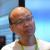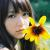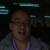## 一个很简单的算法问题

00public static Integer[] transformCloumnID(String[] input) {
Integer[] output = new Integer[input.length];
for (int i = 0; i < input.length; i++) {
char c[] = input[i].toUpperCase().toCharArray();
if(c.length < 1){
output[i] = 0;

}
else {
int length = c.length;
int sum = 0;
for(int j=0;j<c.length;j++){

sum += (((int)c[j])-64) * Math.pow(26,length-j-1);
}
output[i] = sum - 1;
}
}
return output;

}

0
0#### 引用来自#5楼“红薯”的帖子

char 也就是 int

sum += (c[j]- '@') * Math.pow(26,length-j-1);会不会很帅呢

0#### 引用来自#5楼“红薯”的帖子

char 也就是 int

sum += (c[j]- '@') * Math.pow(26,length-j-1);会不会很帅呢

0#### 引用来自#5楼“红薯”的帖子

char 也就是 int

sum += (c[j]- '@') * Math.pow(26,length-j-1);会不会很帅呢

0#### 引用来自#5楼“红薯”的帖子

char 也就是 int